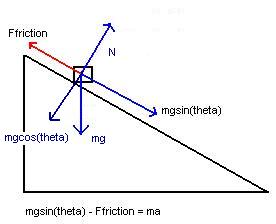# An extreme skier, starting from rest, coasts down a mountainthat makes an angle 25.0^\circ with the horizontal. The coefficient of kinetic friction beLine 2020-11-03 Answered
An extreme skier, starting from rest, coasts down a mountainthat makes an angle ${25.0}^{\circ }$ with the horizontal. The coefficient of kinetic friction between her skis and the snow is 0.200. She coasts for a distance of 11.9 m before coming to the edge of a cliff. Without slowing down, she skis offthe cliff and lands down hill at a point whose vertical distance is 4.20 m below the edge. How fast is she going just before she lands?
You can still ask an expert for help

• Questions are typically answered in as fast as 30 minutes

Solve your problem for the price of one coffee

• Math expert for every subject
• Pay only if we can solve itfalhiblesw

First find the skier's final speed when she clears the cliff:
Before we can use the equation ${v}_{f}^{2}={v}_{o}^{2}+2ad$ we need her acceleration.
If you drew a free body diagram, you should come up with thefollowing equation:
$mg\mathrm{sin}\theta -{F}_{\mathfrak{i}ction}=ma$${F}_{\mathfrak{i}ction}=mg\mathrm{cos}\theta$
$mg\mathrm{sin}\theta -mg\mathrm{cos}\theta =ma$
the masses cancel and you have:
$a=g\left(\mathrm{sin}\theta -\mathrm{cos}\theta \right)=9.81\left(\mathrm{sin}\left(25\right)-\mathrm{cos}\left(25\right)\cdot 0.2\right)=2.37\frac{m}{{s}^{2}}$
Now use acceleration to find her final velocity before leavingthe cliff:
${v}_{o}=0$

$d=11.9m$
${v}_{f}=\sqrt{\left(2\right)\left(9.81\right)\left(11.9\right)}=7.51\frac{m}{s}$
She leaves the cliff at this speed at 25 deg to the horizontal, so
${V}_{\otimes }=7.51\cdot \mathrm{cos}\left(25\right)=6.80\frac{m}{s}$

Now we just need to find ${V}_{f}x$ and ${V}_{f}v$
, and then take their magnitude to get the final answer.